Common Core: 2nd Grade Math : Determine Even or Odd Number of Objects: CCSS.Math.Content.2.OA.C.3

Example Questions

← Previous 1 3

Example Question #1 : Determine Even Or Odd Number Of Objects: Ccss.Math.Content.2.Oa.C.3

Is there an even or an odd number of dots below?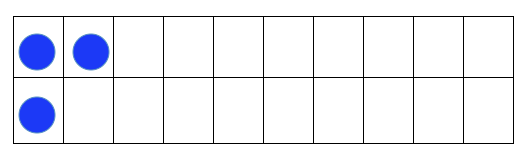Odd

Both

Even

Neither

Odd

Explanation:

When trying to decide if we have an even or an odd number of items, we can put the items in pairs of two. If each item is in a pair, then we have an even number of items. If there is one item that isn't in a pair, then we have an odd number of items.

In this case, we have one dot not in a pair, so we have an odd number of dots.

Example Question #2 : Determine Even Or Odd Number Of Objects: Ccss.Math.Content.2.Oa.C.3

Is there an even or an odd number of dots below?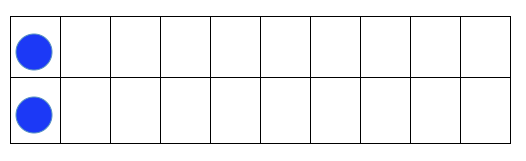Both

Odd

Neither

Even

Even

Explanation:

When trying to decide if we have an even or an odd number of items, we can put the items in pairs of two. If each item is in a pair, then we have an even number of items. If there is one item that isn't in a pair, then we have an odd number of items.

In this case, our dots are in a pair of two, so we have an even number of dots.

Example Question #3 : Determine Even Or Odd Number Of Objects: Ccss.Math.Content.2.Oa.C.3

Is there an even or an odd number of dots below?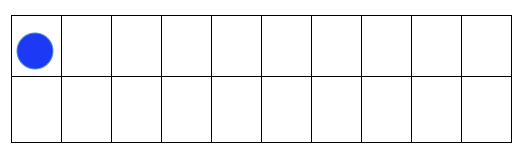Even

Neither

Odd

Both

Odd

Explanation:

When trying to decide if we have an even or an odd number of items, we can put the items in pairs of two. If each item is in a pair, then we have an even number of items. If there is one item that isn't in a pair, then we have an odd number of items.

In this case, we have one dot. This dot is not in a pair, so we have an odd number of dots.

Example Question #4 : Determine Even Or Odd Number Of Objects: Ccss.Math.Content.2.Oa.C.3

Is there an even or an odd number of dots below?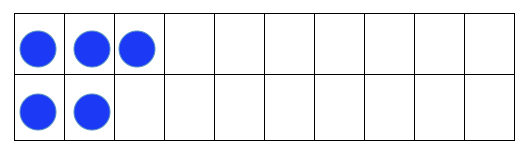Both

Neither

Even

Odd

Odd

Explanation:

When trying to decide if we have an even or an odd number of items, we can put the items in pairs of two. If each item is in a pair, then we have an even number of items. If there is one item that isn't in a pair, then we have an odd number of items.

In this case, we have one dot not in a pair, so we have an odd number of dots.

Example Question #5 : Determine Even Or Odd Number Of Objects: Ccss.Math.Content.2.Oa.C.3

Is there an even or an odd number of dots below?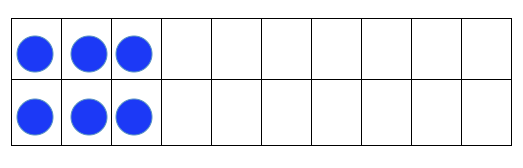Neither

Both

Odd

Even

Even

Explanation:

When trying to decide if we have an even or an odd number of items, we can put the items in pairs of two. If each item is in a pair, then we have an even number of items. If there is one item that isn't in a pair, then we have an odd number of items.

In this case, our dots are in pairs of two, so we have an even number of dots.

Example Question #6 : Determine Even Or Odd Number Of Objects: Ccss.Math.Content.2.Oa.C.3

Is there an even or an odd number of dots below?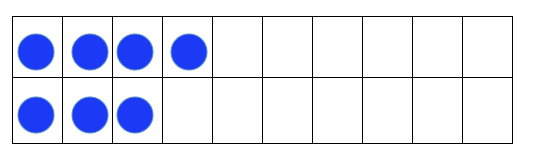Odd

Even

Neither

Both

Odd

Explanation:

When trying to decide if we have an even or an odd number of items, we can put the items in pairs of two. If each item is in a pair, then we have an even number of items. If there is one item that isn't in a pair, then we have an odd number of items.

In this case, we have one dot not in a pair, so we have an odd number of dots.

Example Question #7 : Determine Even Or Odd Number Of Objects: Ccss.Math.Content.2.Oa.C.3

Is there an even or an odd number of dots below?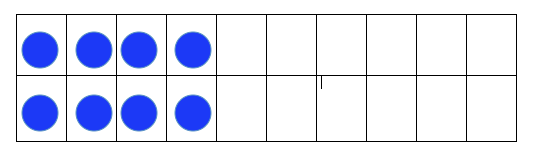Both

Neither

Odd

Even

Even

Explanation:

When trying to decide if we have an even or an odd number of items, we can put the items in pairs of two. If each item is in a pair, then we have an even number of items. If there is one item that isn't in a pair, then we have an odd number of items.

In this case, our dots are in pairs of two, so we have an even number of dots.

Example Question #8 : Determine Even Or Odd Number Of Objects: Ccss.Math.Content.2.Oa.C.3

Is there an even or an odd number of dots below?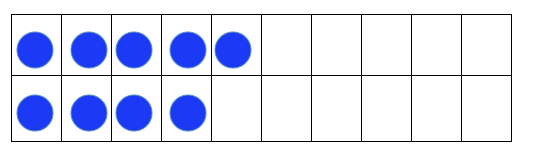Both

Even

Odd

Neither

Odd

Explanation:

When trying to decide if we have an even or an odd number of items, we can put the items in pairs of two. If each item is in a pair, then we have an even number of items. If there is one item that isn't in a pair, then we have an odd number of items.

In this case, we have one dot not in a pair, so we have an odd number of dots.

Example Question #9 : Determine Even Or Odd Number Of Objects: Ccss.Math.Content.2.Oa.C.3

Is there an even or an odd number of dots below?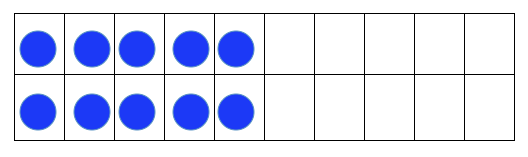Both

Even

Odd

Neither

Even

Explanation:

When trying to decide if we have an even or an odd number of items, we can put the items in pairs of two. If each item is in a pair, then we have an even number of items. If there is one item that isn't in a pair, then we have an odd number of items.

In this case, our dots are in pairs of two, so we have an even number of dots.

Example Question #10 : Determine Even Or Odd Number Of Objects: Ccss.Math.Content.2.Oa.C.3

Is there an even or an odd number of dots below?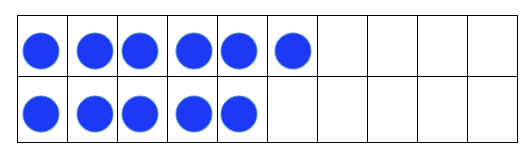Neither

Both

Odd

Even